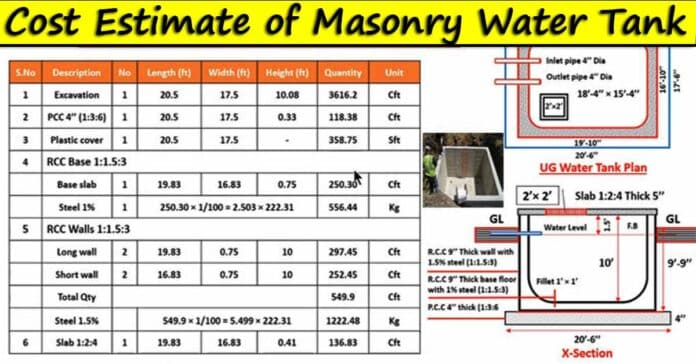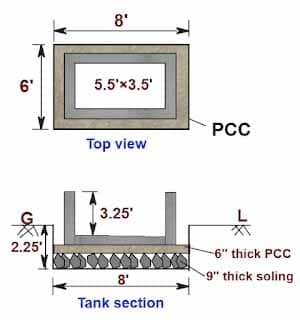# How to Calculate the Estimate of Masonry Water Tank | Cost Estimation of Brick Masonry Water Tank

In this Article today we will talk about the How To Calculate the Estimate of Masonry Water Tank | Rectangular Water Tank Estimate | Cost Estimate of Brick Masonry Water Tank | Water Tank Calculator | Estimate of Water Tank | How to Find the Quantity of Water Tank | Underground Water Tank Estimate | Material Estimate of Water Tank | Types of Water Tanks

## Masonry Water Tank:

Dating back to the early days of civilization, water storage facilities have been a part of the construction industry forever. Today there are many smaller pre-built water tanks available in the market, but more often than not we have to custom-build our water storages. To build one of them, you have to know about the amount of construction materials it needs. Today, we will give you a sample materials estimation of an Masonry water tank. Rectangular Water Tank Estimate

Depending upon the usage and scenario, there can be many types of water storage tanks. Especially in dry areas, you have to do some serious thinking about how exactly would you want to store your water. Because you need a consistent supply of water without getting it wasted or overdrawn. Water Tank Calculator

### Types of water tanks

Here are some common varieties of water storage tanks we can find: Water Tank Calculator

### Drinking water tank

These are either small portable tanks, or fixed large vertical tanks. Either are generally cylindrical in shape. Sometimes filtering equipment are fitted on the outlet of the tanks. They require regular maintenance and cleaning. How to Find the Quantity of Water Tank

### Fire suppression tank

Some builders employ a large tank (or a system of them) connected to the sprinkler system in the building. These tanks aren’t generally used but kept full as backup.

### Irrigation tank

Depending upon if you want to irrigate your field or water your plants, the irrigation tanks can be large or small. They don’t require filtration systems and so much regular cleanup. These are connected to the irrigation channels via regulator or to the garden sprinklers or hose. Underground Water Tank Estimate

### Waste water storage

These are generally large, underground vaults used to hold sewage. They may be connected to the drainage lines or may not. Types of Water Tank

## How to Calculate the Estimate of Masonry Water Tank:

Let us estimate the volume of materials in the brick masonry water tank as shown below: Material Estimate of Water Tank

### Given data:

Size of the tank = 5.5 ft. × 3.5 ft.

Depth of the tank = 3.25 ft.

Masonry wall thickness = 9″ = 0.75 ft.

#### The volume of the tank

= L × B × H

= 5.5 ft. × 3.5 ft. × 3.25 ft.

= 62.56 cu ft.

1771 liters.

( As 1 cu ft. = 28.315 liters.)

Now let us calculate the vol. & qty. of different items of the water tank.#### 1. Excavation:

The volume of excavation for the tank

= [L × B × H]

= [ 8 ft. × 6 ft. ×2.25 ft.]

108 cu ft. Types of Water Tank

#### 2. Rubble soling:

The volume of rubble soling

= [L × B × H]

= [8 ft. × 6 ft. × 0.75 ft.]

36 cu ft.

#### 3. Bed PCC:

The volume of bed PCC

= [L × B × H]

= [8 ft. × 6 ft. × 0.5 ft.]

24 cu ft.

#### 4. Brick masonry:

Let us calculate the masonry vol. by the longwall & short wall method.

The vol. of brick masonry

= [(2nos. × vol. of longwall) + (2nos. × vol. of the short wall)]

= [ ( 2nos. × L × T × H  of longwall) + ( 2nos. × L × T × H of a short wall )]

= [ ( 2nos. × 7 ft. × 0.75 ft. × 4 ft.) + ( 2nos. × 3.5 ft. × 0.75 ft. × 4 ft. )]

= [ ( 42 cu ft. ) + ( 21 cu ft. )]

63 cu ft.

#### 5. Plastering:

Let us make internal & external plaster in a 1:4 ratio having 12mm. thickness.

##### 5a. Internal plaster:

The vol. of internal plaster

= [(2nos. × vol. of longwall plaster) + (2nos. × vol. of the short wall plaster)]

= [ ( 2nos. × L × T × H  of longwall plaster) + ( 2nos. × L × T × H of a short wall plaster ) ]

= [ ( 2nos. × 7 ft. × 0.039 ft. × 3.25 ft.) + ( 2nos. × 3.5 ft.  × 0.039 ft. × 3.25 ft. )] ( 12mm. = 0.039 ft.)

= [ ( 1.7745 cu ft. ) + ( 0.887 cu ft. )]

2.662 cu ft.

##### 5b. Top surface & internal base plaster:

The vol. of top & base plaster

= [ ( L × W × T of top masonry surface) + (  L × W × T  of base plaster )]

Here, L = [(2nos. × 8 ft.) + (2 nos. × 3.5 ft.)] = 23 ft.

=  [ ( 23 ft. × 0.75 ft. × 0.039 ) + (  5.5 ft. × 3.5 ft. × 0.039  )]

=  [( 0.67cu ft.) + ( 0.75 cu ft. )]

1.42 cu ft.

##### 5c. External plaster:

The vol. of external plaster

= [ ( 2nos. × L × T × H  of longwall plaster) + ( 2nos. × L × T × H of a short wall plaster ) ]

= [ ( 2nos. × 8 ft. × 0.039 ft. × 4 ft.) + ( 2nos. × 5 ft.  × 0.039 ft. × 4 ft. )] ( 12mm. = 0.039 ft.)

= [ ( 2.496 cu ft. ) + ( 1.56 cu ft. )]

4.056 cu ft.

The total vol. of plaster

= [ 5a. + 5b. + 5c.]

= [ 2.662 cu ft. + 1.42 cu ft. +4.056 cu ft.]

8.138 cu ft.

Now, let us calculate the cost of the masonry water tank as shown below:

Let us calculate the water tank cost in the table format.

Thumb rule:

The total cost of water tank = INR 24,578/-

The volume of the tank = 1771 liters.

The cost of masonry water tank/liter

= [ total water tank cost ÷ vol. in liters]

= [ 24,578  ÷ 1771]

13.87 / liter.

## Conclusion:

Full article on How To Calculate the Estimate of Masonry Water Tank | Rectangular Water Tank Estimate | Cost Estimate of Brick Masonry Water Tank | Water Tank Calculator | Estimate of Water Tank | How to Find the Quantity of Water Tank | Underground Water Tank Estimate | Material Estimate of Water Tank | Types of Water Tanks. Thank you for the full reading of this article in “The Civil Engineering” platform in English. If you find this post helpful, then help others by sharing it on social media. If any formula of BBS is missing from this article please tell me in comments.